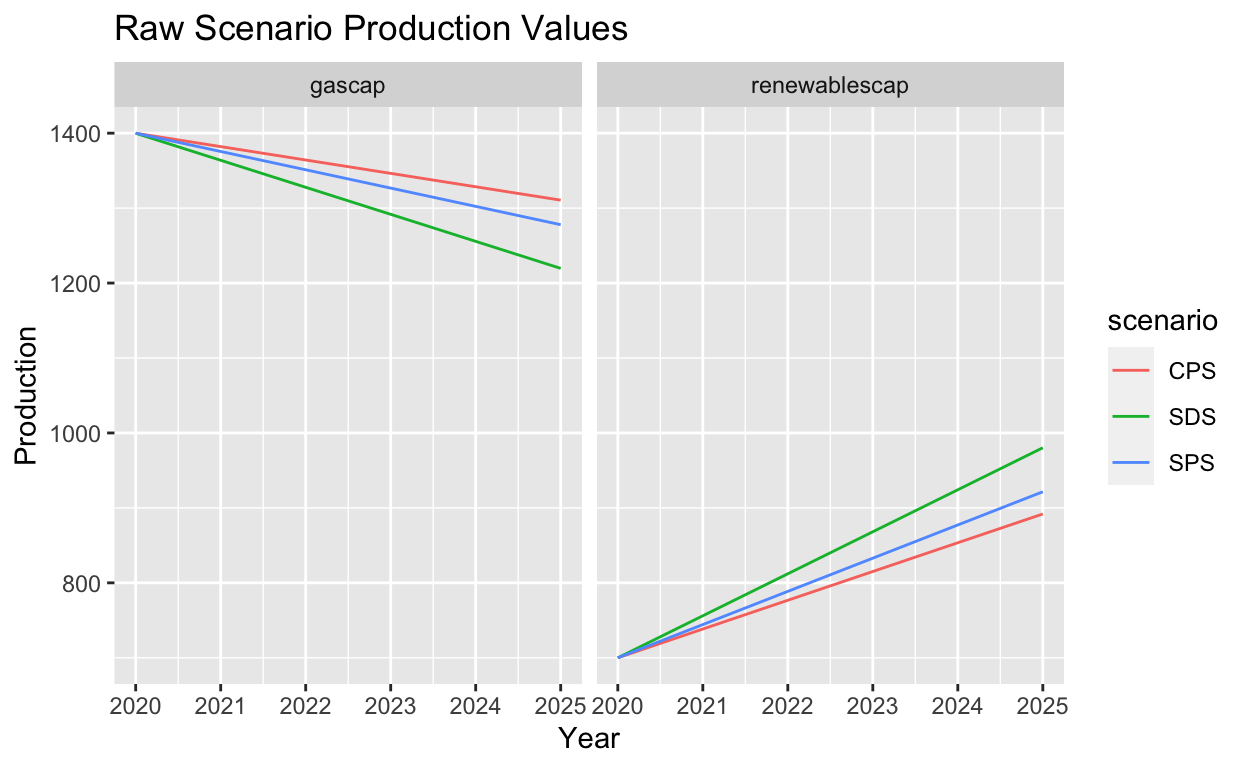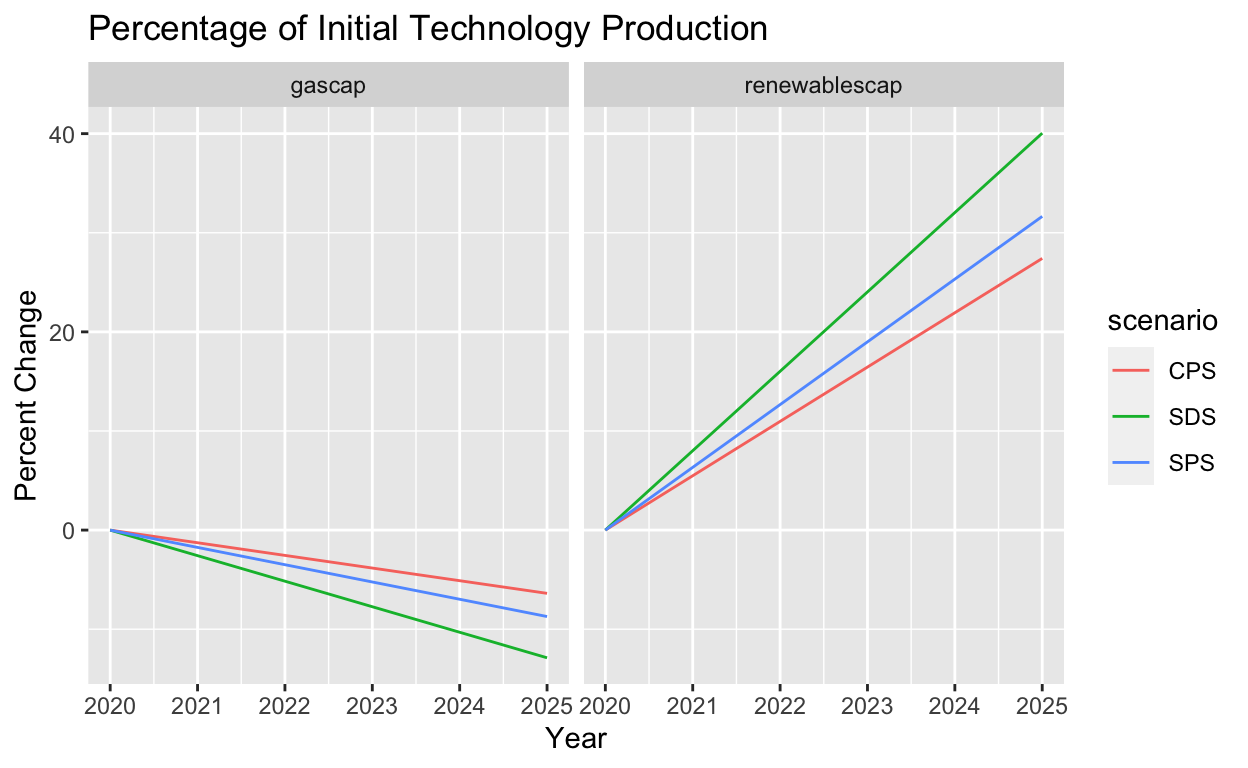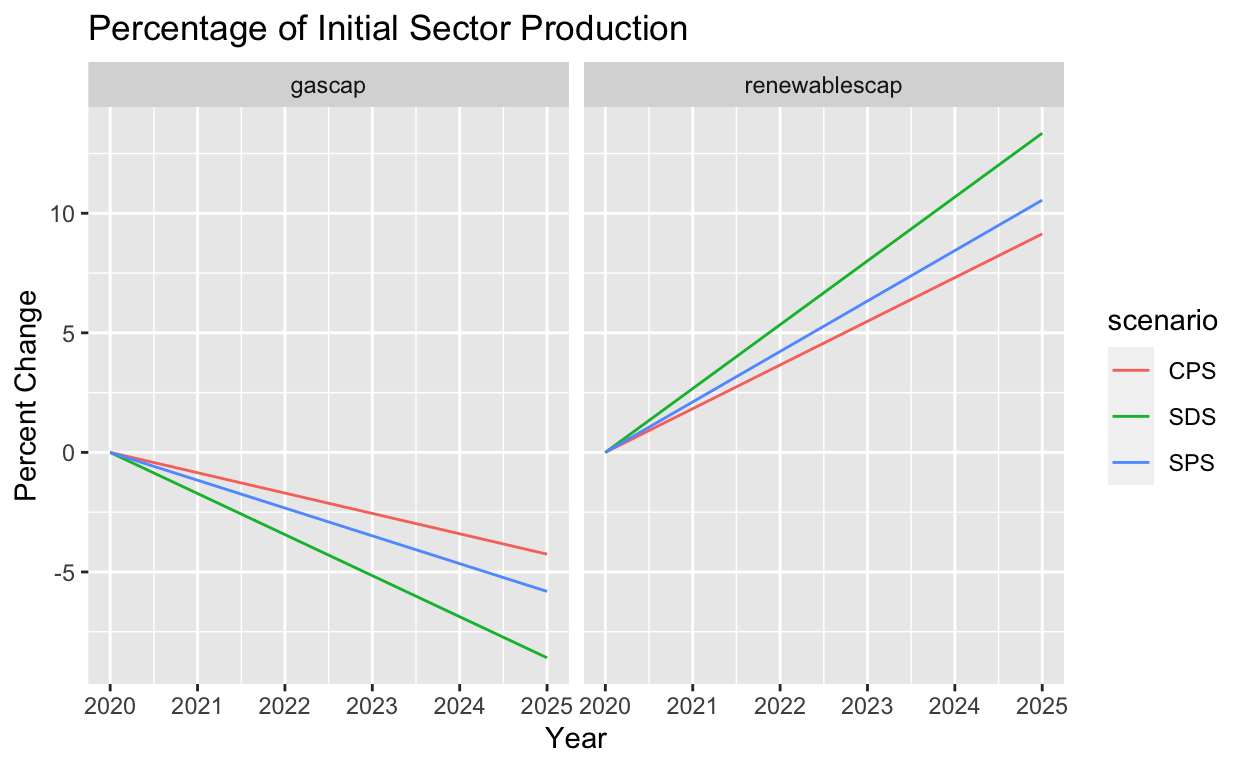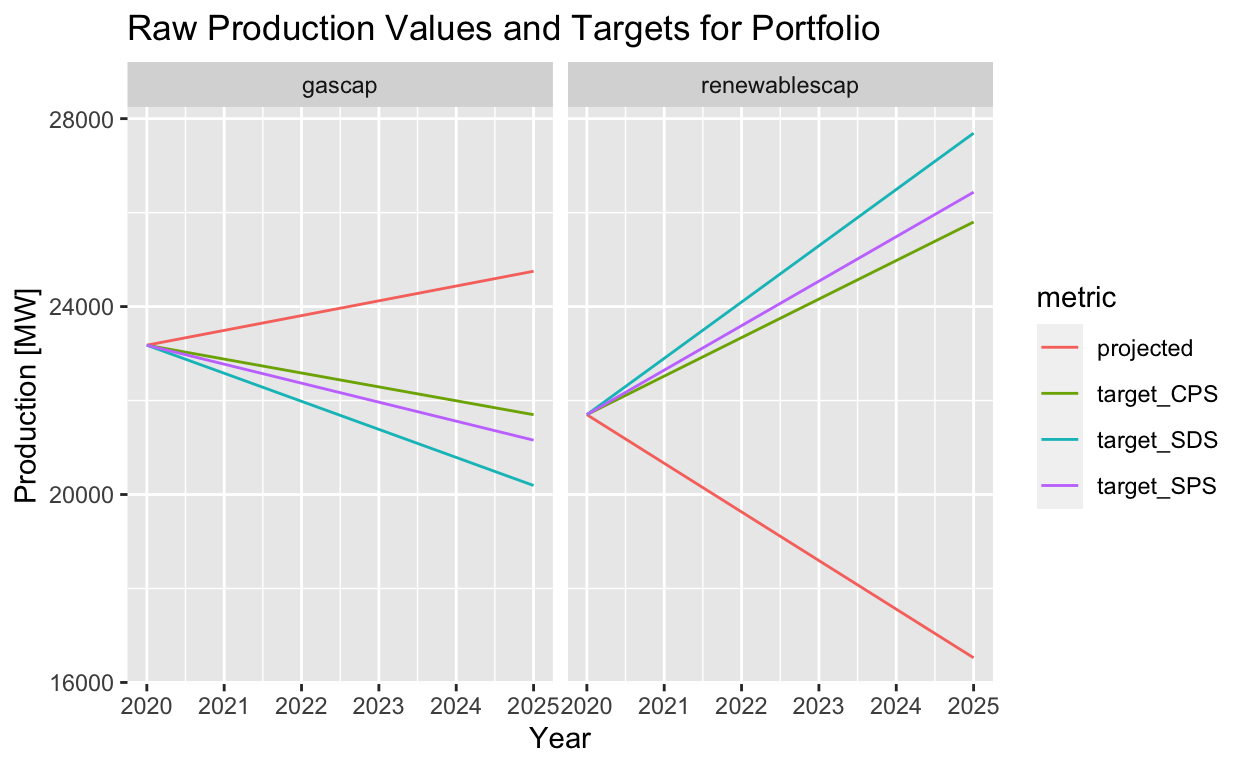# Market Share Target Setting Methodology - Calculation and Plotting

A comprehensive explanation of the PACTA market share target setting methodology, and the associated difficulties when it comes to interpretation and plotting.

Jackson Hoffart https://github.com/jdhoffa
2022-05-02

A core component of the PACTA methodology is the ability to allocate macro-economic efforts to micro-economic actors. More specifically, PACTA takes climate change mitigation scenarios, which provide production pathways for various sectors and technologies, and allocates these efforts to individual companies, and/or financial portfolios.

In this note, we will:

• Discuss PACTA’s current target-setting methodology (Technology- and Sector-Market Share)
• Explain the rationale and short-comings of the current volume trajectory plots
• Propose a new plotting method that aims to address these short-comings.

This note assumes a basic understanding of the PACTA methodology.

# Target-Setting with PACTA

We will use fake data throughout this note to illustrate examples.

First, let’s define a couple of fake climate scenarios. We will need to have an example of an increasing scenario, such as renewable energy production (hereby referred to as “renewables” or “renewablescap”), and a decreasing scenario, such as gas-fired energy production (hereby referred to as “gas” or “gascap”).

These scenarios will start at arbitrary values, and have varying degrees of ambition.A crucial function of the PACTA methodology is to allocate a portion of the scenario production to different companies, and financial portfolios.

To do so, PACTA applies two different methods, depending on if the technology is projected to increase (as is the case with low-carbon technologies, such as renewables) or if it is projected to decrease (as is the case with high-carbon technologies, such as gas).

## High-Carbon Technologies - Technology Market Share

It is straight-forward to calculate the percent-change of any technology’s production at time $$t$$, as a percentage of the initial value at $$t_0$$:

$\left(\frac{s_i(t)-s_i(t_0)}{s_i(t_0)}\right)*100$

where $$s(t)$$ is the scenario’s production, for some technology, at time $$t$$.

Note that this reduces to:

$\left(\frac{s_i(t)}{s_i(t_0)} - 1\right)*100$

For reason’s that will become clear later, we will refer to this as the technology-market share.

We can transform the scenarios defined above, and plot this value:From this value, we can set a target for a portfolio as:

$p_i^{target}(t) = p_i(t_0) + p_i(t_0)*\left(\frac{s_i(t)-s_i(t_0)}{s_i(t_0)}\right)$

where $$p_i(t)$$ is the portfolio’s actual production in technology $$i$$ and $$p_i^{target}$$ is the portfolio’s calculated target production.

## Low-Carbon Technologies - Sector Market Share

Rather than calculating the percent change as a percentage of the initial technology value, we might also calculate

$\left(\frac{s(t) - s(t_0)}{S(t_0)}\right)*100$ where $$S(t)$$ now refers to the scenario’s production in the entire sector, at time $$t$$. We will refer to this as the sector-market share.

Plotting this value yields:Similarly, we can use this value to calculate a target as:

$p_i^{target}(t) = p_i(t_0) + P(t_0)*\left(\frac{s(t) - s(t_0)}{S(t_0)}\right)$ where $$P(t_0)$$ is the portfolio’s production in the entire sector.

# Rationale for Having Two Approaches

This begs the question, why two approaches? We will illustrate with an example.

## Technology-Market Share Doesn’t Work for Low-Carbon

First, imagine a portfolio that is exposed only to a high-carbon technology, such as Gas-fired Power. Lets say that $$p_{gas}(t_0) = 500MW$$ and $$p_{renewables}(t_0) = 0MW$$.

Imagine we used only the technology-market share to calculate targets. The function looks like:

\begin{aligned} p_i^{target}(t) &= p_i(t_0) + p_i(t_0)*\left(\frac{s_i(t)-s_i(t_0)}{s_i(t_0)}\right) \\ &= p_i(t_0) + p_i(t_0)*\left(\frac{s_i(t)}{s_i(t_0)} - 1\right) \\ &= p_i(t_0) * \left(\frac{s_i(t)}{s_i(t_0)}\right) \\ \end{aligned}

for our gas example, this would become: $p_{gas}^{target}(t) = 500MW * \left(\frac{s_i(t)}{s_i(t_0)}\right)$ just the initial gas production, multiplied by the scenario ratio. So long as there are non-zero scenario values, there will be a target for gas production.

However, for renewable power, since $$p_i(t_0) = 0MW$$:

\begin{aligned} p_{renewables}^{target}(t) &= 0MW * \left(\frac{s_i(t)}{s_i(t_0)}\right) \\ &= 0MW \end{aligned} Since we are multiplying by 0, we will never have a target for renewables.

This can be interpreted as follows. The portfolio is only exposed to gas, which is a technology expected to decline in production to curb climate change. Since the portfolio currently has NO market share in renewables, it won’t be expected to build-out renewables (following the technology-market share approach). Thus, a target calculated in this method would suggest that a portfolio simply declines it’s production entirely, without transitioning to low-carbon technologies. This doesn’t make sense, since eventually the companies would decline production so much that they fail.

Now, if we were to use instead the sector market share for renewables, we see:

\begin{aligned} p_{renewables}^{target}(t) &= p_{renewables}(t_0) + P(t_0)*\left(\frac{s(t) - s(t_0)}{S(t_0)}\right) \\ &= 0MW + 500MW * \left(\frac{s(t) - s(t_0)}{S(t_0)}\right) \end{aligned}

Though the portfolio doesn’t have any exposure to the renewables technology, it does have exposure to the power sector. Thus, if we allocate the desired renewable growth scenario as a percentage of the overall sector, we will end up with a non-zero target.

## Sector-Market Share Doesn’t Work for High-Carbon

The natural question is then, why can’t we just apply the sector-market share equation to high-carbon technologies?

Let’s explore with another example. For now, we will just use the equations. In particular, let’s explore conditions such that the target calculated is negative (note: this should not happen, as it has no physical interpretation). If we start with the Sector-Market Share equation:

$p_i^{target}(t) = p_i(t_0) + P(t_0)*\left(\frac{s(t) - s(t_0)}{S(t_0)}\right)$ For our negative target boundary-case, this becomes:

$0 > p_i(t_0) + P(t_0)*\left(\frac{s_i(t) - s_i(t_0)}{S(t_0)}\right)$

Putting all portfolio-related terms on the LHS, and scenario-related terms on the RHS, and handling signs a bit, we get:

$\frac{p_i(t_0)}{P(t_0)} < \frac{s_i(t_0)-s_i(t)}{S(t_0)}$

It is quite easy to satisfy this condition. Consider the fake scenario we defined above:

Scenario Production Values
scenario technology Year Production
SDS gascap 2020 1400.0000
SDS renewablescap 2020 700.0000
SDS gascap 2025 1219.6053
SDS renewablescap 2025 980.3345

i.e. $s_{gas}(t_0) = 1400MW$, $$s_{gas}(2025) \approx 1220MW$$ and $$S(t_0) \approx 2100MW$$. Now suppose we have a portfolio that has a small exposure to gas production, let’s say $$10MW$$, and a large exposure to renewable production, let’s say $$1000MW$$, i.e.. $$p_{gas}(t_0) = 10MW$$ and $$P(t_0) = 1100MW$$, then this portfolio’s gas target becomes:

\begin{aligned} p_{gas}^{target}(t) &= p_{gas}(t_0) + P(t_0)*\left(\frac{s_{gas}(t) - s_{gas}(t_0)}{S(t_0)}\right) \\ &= 10MW + 1100MW*\left(\frac{1220MW - 1400MW}{2100MW}\right) \\ &\approx -84.3 MW \end{aligned}

Specific values were chosen to illustrate the point, but as shown, this condition can be easily met with arbitrary input values. This is a problem for multiple reasons. First, of all, negative production targets have no physical interpretation. Second, since there is no physical way to produce “negative” energy, we are effectively allocating impossible scenario ambitions. Therefor, if we were to continue forward setting targets like this, we would not be adequately accounting for the necessary production ramp-down that the scenario projects.

Even if we were to just set negative targets to 0, there would be scenario ambition that does not get considered, and our targets would not adequately limit CO2 emissions to the proposed carbon budget.

# Plotting Results: Volume Trajectory Charts

Now that we have explained the target-setting methodology in detail, we can look into what the output of a PACTA run might look like for some arbitrary loanbook. For the sake of simplicity, we will assume a loanbook with only one exposure to some company that has both Gas and Renewables assets.

Sample PACTA Output
Sector Technology Year Region Scenario Source Metric Production Technology Share scope percentage_of_initial_production_by_scope
power gascap 2020 global demo_2020 projected 23178.46 0.5164751 technology 0.00000000
power gascap 2020 global demo_2020 target_CPS 23178.46 0.5164751 technology 0.00000000
power gascap 2020 global demo_2020 target_SDS 23178.46 0.5164751 technology 0.00000000
power gascap 2020 global demo_2020 target_SPS 23178.46 0.5164751 technology 0.00000000
power gascap 2025 global demo_2020 projected 24752.64 0.5996650 technology 0.06791575
power gascap 2025 global demo_2020 target_CPS 21699.01 0.4568243 technology -0.06382884
power gascap 2025 global demo_2020 target_SDS 20191.84 0.4216959 technology -0.12885334
power gascap 2025 global demo_2020 target_SPS 21156.77 0.4445496 technology -0.08722284
power renewablescap 2020 global demo_2020 projected 21699.72 0.4835249 sector 0.00000000
power renewablescap 2020 global demo_2020 target_CPS 21699.72 0.4835249 sector 0.00000000
power renewablescap 2020 global demo_2020 target_SDS 21699.72 0.4835249 sector 0.00000000
power renewablescap 2020 global demo_2020 target_SPS 21699.72 0.4835249 sector 0.00000000
power renewablescap 2025 global demo_2020 projected 16524.81 0.4003350 sector -0.11531011
power renewablescap 2025 global demo_2020 target_CPS 25800.68 0.5431757 sector 0.09137978
power renewablescap 2025 global demo_2020 target_SDS 27690.62 0.5783041 sector 0.13349261
power renewablescap 2025 global demo_2020 target_SPS 26434.70 0.5554504 sector 0.10550757
power gascap 2020 global demo_2020 corporate_economy 1195546.70 0.1130896 technology 0.00000000
power gascap 2025 global demo_2020 corporate_economy 1218539.70 0.1141743 technology 0.01923221
power renewablescap 2020 global demo_2020 corporate_economy 1598339.46 0.1511907 sector 0.00000000
power renewablescap 2025 global demo_2020 corporate_economy 1483450.28 0.1389958 sector -0.01086764

First, let’s just plot the absolute production values:## Volume Trajectory with Technology-Market Share

Now, let’s see what we actually plot in the PACTA volume trajectory plots, using r2dii.plot, first for a decreasing technology (Gas).